# Delaunay Mesh Refinement

## Application Description

This benchmark is an implementation of the algorithm described by Kulkarni et al. . The application produces a guaranteed quality Delaunay mesh, which is a Delaunay triangulation with the additional constraint that no angle in the mesh be less than 30 degrees. The benchmark takes as input a Delaunay triangulation of a 2D region, and produces a refined mesh that satisfies the quality constraint. The data parallelism in this algorithm arises from the worklist of badly shaped triangles that must be "fixed."

 Milind Kulkarni, Keshav Pingali, Bruce Walter, Ganesh Ramanarayanan, Kavita Bala and L. Paul Chew. Optimistic Parallelism Requires Abstractions. In Proceedings of the ACM Conference on Programming Languages Design and Implementation (PLDI), 211 - 222, June 2007.

## Algorithm

A 2D Delaunay mesh is a triangulation of a set of points with the following property: the circumcircle of any triangle in the mesh must contain no other point from the mesh. A refined Delaunay mesh is a Delaunay mesh with the additional constraint that no triangle have an angle less than 30 degrees. The algorithm takes an input Delaunay mesh (e.g., the top mesh in Figure 1), which may contain triangles that do not meet the quality constraints (called bad triangles, marked in red in Figure 1), and produces a refined mesh by iteratively re-triangulating the affected portions of the mesh.

The algorithm is initialized with a worklist of all the bad triangles in the input mesh. In each step, the refinement procedure (i) picks a bad triangle from the worklist, (ii) collects the affected triangles in the neighborhood of that bad triangle (called the cavity, shown in blue in Figure 1), and (iii) re-triangulates the cavity (creating the new green triangles in Figure 1). If this re-triangulation creates new badly-shaped triangles in the cavity, they are processed as well until all bad triangles have been eliminated from the mesh. The order in which the bad triangles are processed is irrelevant because all orders lead to a valid refined mesh, so this is an unordered algorithm.

In more detail, the algorithm proceeds as follows (pseudocode is provided in Figure 2). After reading in the input mesh (line 1), a worklist is initialized with the bad triangles in the mesh (line 2). For each bad triangle, a cavity is created (line 4) and expanded to encompass the required neighboring triangles (line 5). The algorithm then determines the new triangles that should be created (line 6) and updates the original mesh by removing the old triangles and adding the new triangles (line 7). Since the order of processing is irrelevant in this algorithm, the foreach in line 3 iterates over an unordered set.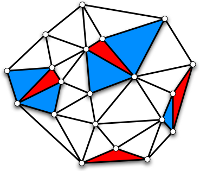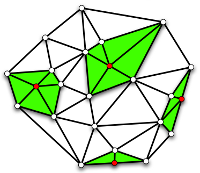Figure 1: Delaunay mesh refinement example.

 1 2 3 4 5 6 7 8 9 Mesh m = /* read input mesh */; Workset ws = new Workset(m.getBad()); foreach (Triangle t : ws) { Cavity c = new Cavity(t); c.expand(); c.retriangulate(); m.updateMesh(c); ws.add(c.getBad()); }

Figure 2: Pseudocode for Delaunay mesh refinement.

## Data Structures

There are two key data structures used in Delaunay mesh refinement:

Unordered Set
The worklist used to hold the bad triangles is represented as an unordered set. The triangles can be processed in any order.
Graph
The mesh is represented as a graph. Triangles in the mesh are represented as nodes in the graph, and triangle adjacency is represented by edges between nodes.

## Demo

A demo of Delaunay mesh refinement

## Parallelism

The active nodes in Delaunay mesh refinement are the bad triangles. The algorithm can be parallelized by processing multiple bad triangles simultaneously. Because the neighborhood of a bad triangle is its cavity, this may result in significant parallelism if the triangles are far enough apart so that their cavities do not overlap (as in Figure 1, where all of the bad triangles can be processed in parallel). Conflicts occur when cavities overlap. This is manifested in the algorithm by multiple update operations (line 7) attempting to manipulate the same triangles.

Figure 3 shows the available parallelism of Delaunay mesh refinement for a small input. Delaunay mesh refinement is a graph refinement code because each retriangulated cavity replaces an existing subgraph with a new subgraph that contains more nodes. Thus, as the graph becomes bigger, the likelihood of two cavities overlapping decreases and the available parallelism increases accordingly until the algorithm runs out of work.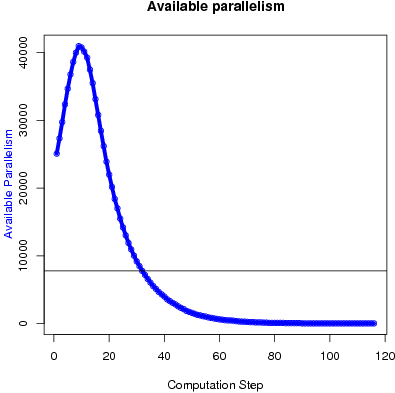Figure 3: Available parallelism in Delaunay mesh refinement for random input of 549,998 triangles of which 261,100 are initially bad.

## Performance

Figures 4 and 5 show the execution time in seconds and self-relative speedup (speedup with respect to the single thread performance), respectively, of this algorithm. The input is a mesh generated from triangulation of one million randomly generated points. The initial mesh has 2,000,004 total triangles of which 949,587 are bad. On the same input, the Triangle program takes about 12 seconds.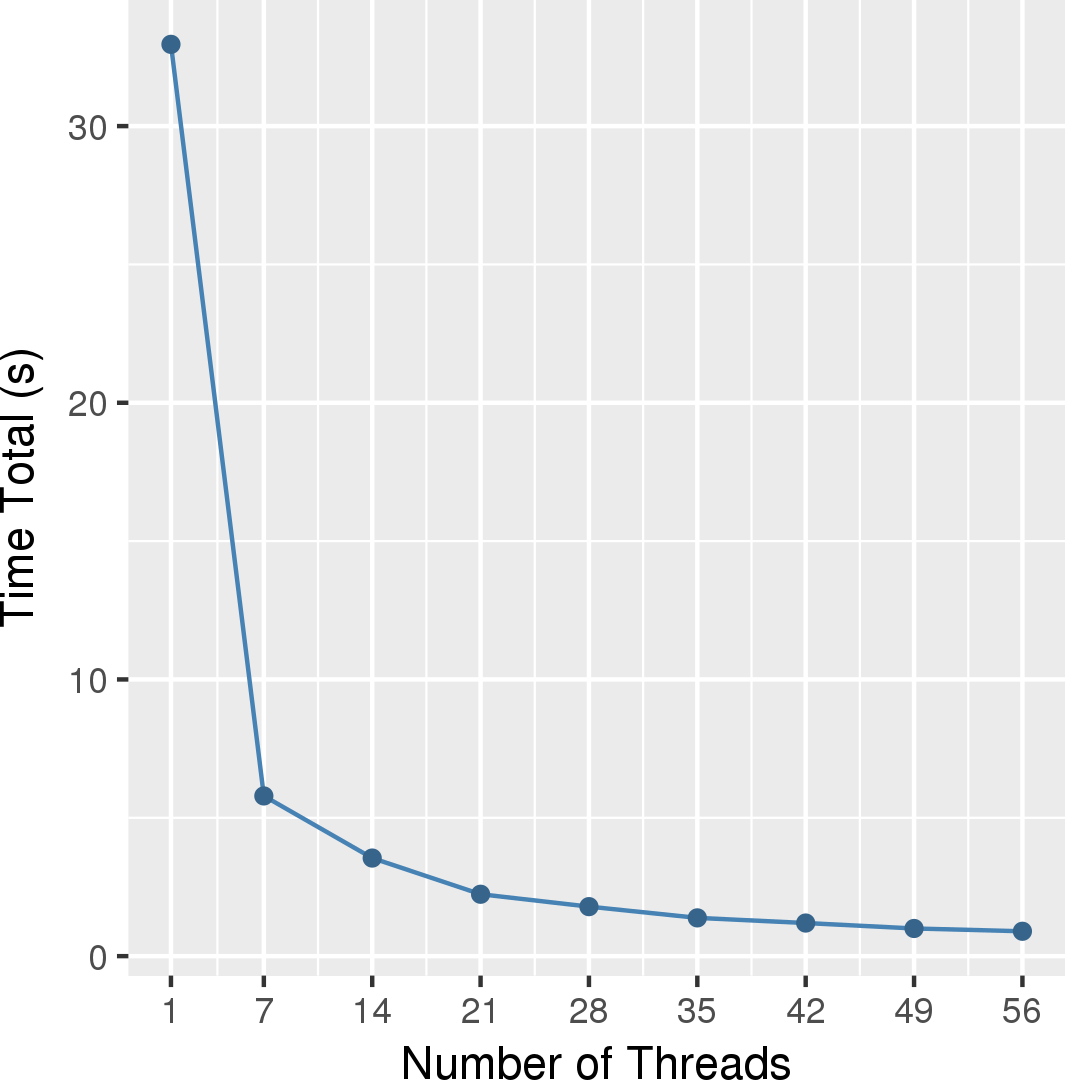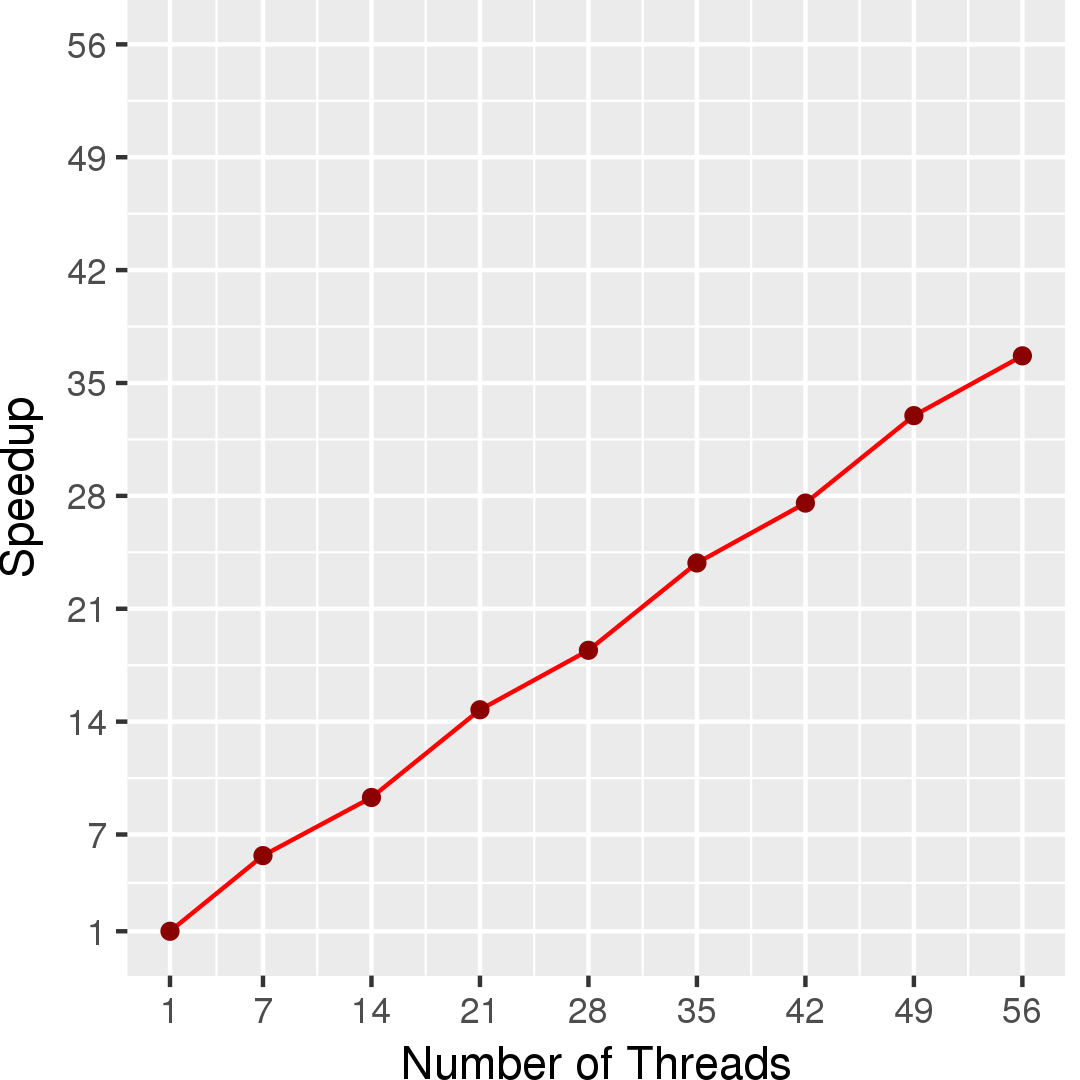## Machine Description

Performance numbers are collected on a 4 package (14 cores per package) Intel(R) Xeon(R) Gold 5120 CPU machine at 2.20GHz from 1 thread to 56 threads in increments of 7 threads. The machine has 192GB of RAM. The operating system is CentOS Linux release 7.5.1804. All runs of the Galois benchmarks used gcc/g++ 7.2 to compile.